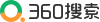创造营2021[(2021.11.1)] 深耕细研踏歌行 齐心协力共提升

[(2021.11.1)] 精诚团结共发展，教学研讨同提升

[(2021.11.1)] 潜心专研，携手互助，共同成长！

[(2021.11.1)] 同课异构展风采，教学研讨促成长

[(2021.10.15)] 国庆新民俗，八中开好头

[(2021.10.15)] 爱国 爱校 爱学习――八中东校区第一次表彰大..

[(2021.10.2)] 军训生涯，不负韶华――驻马店市第八中学新生..

[(2021.10.2)] 更上层楼山水阔 无边光景一时新

[(2021.10.2)] 少年强则国强，吾辈当自强

[(2021.7.19)] 一路同行，一路感恩！

[(2021.7.19)] 青春拂晓 圆梦今朝―2021毕业典礼

[(2021.6.1)] 红旗飘扬党百年，诗词盛会颂华章

[(2021.5.23)] 特色办学立潮头，教研教改八中行

[(2021.5.23)] 同读百年党史，悦享万卷书香

[(2021.5.1)] 共商共研 精准备考

(2015.4.23) 品味橡皮章[图]

(2013.5.2) 理化生实验课[图]

(2013.5.2) 理化生实验[图]

(2013.5.2) 理化生实验[图]

(2013.5.2) 理化生实验[图]

(2013.4.27) 纪念革命烈士 弘扬民族精神主题班会[图]

(2013.4.27) 美术社团活动[图]

(2013.4.27) 美术社团活动[图]

(2013.4.27) 雷锋精神主题班会[图]

(2013.4.26) 建团九十周年手抄报[图]

(2013.4.26) 收看感动中国刘伟事迹[图]

(2013.4.26) 收看禁毒知识片[图]

(2013.4.26) 艺术节展版[图]

(2013.4.26) 艺术节展版[图]

(2013.4.26) 艺术节展版[图]

(2013.4.26) 艺术节展版[图]

(2013.4.26) 篮球绕杆跑[图]

[(2013.4.26)] 《驻马店日报》报道我校举办英语演讲比赛

[(2013.4.26)] 《驻马店日报》报道我校骨干教师到山东潍坊五..

[(2013.4.26)] 《天中晚报》报道九年级学生备考中招体育考试..

[(2013.4.26)] 《天中晚报》报道我校深入推进课程改革情况

[(2013.4.26)] 《河南经济报》大幅报道我校特色办学纪实

[(2013.4.26)] 《天中晚报》大幅版面报道我校特色课改纪实

[(2013.4.26)] 高效实效，向幸福教育出发------河南卫视走进..

[(2013.4.24)] 《驻马店日报》报道我校举办向道德模范看齐活..

[(2013.4.24)] 《驻马店日报》报道我校交通安全知识讲座

[(2013.4.24)] 中国新闻网深入我校采访

[(2013.4.24)] 河南卫视再次走进八中

[(2013.5.21)] 《七色光》第二期

[(2013.5.21)] 《七色光》第一期

[(2013.5.21)] 《七色光》第三期

[(2013.5.21)] 《七色光》第二期

[(2013.4.24)] 《七色光》第一期

[(2013.4.24)] 驻马店市第八中学校报工作实施细则

[(2013.4.24)] 驻马店市第八中学校报编辑出版的相关规定联系方式

电话：0396-2123817 地址：驻马店文明路与丰泽路交叉口向西50米路北。

学生风采